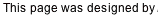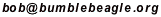## Choosing Three Numbers from 1..10 Using Seven Coin Flips

Nov/15/2009

There are 120 ways to choose three items from a set of 10, and there are 128 results from flipping a two-sided coin seven times. This inspired me to look for a simple-to-remember procedure that would let you make a random choice of three numbers from ten using seven flips of a coin. The procedure will succeed about 94% of the time. The rest of the time it will fail (but after only four flips), requiring you to start over.

Before we start, we divide the numbers into five pairs, {1,6}, {2,7}, {3,8}, {4,9} and {5,10}. The procedure makes use of the diagram below, which can be drawn on a scrap of paper. Coins are placed on the diagram to indicate which numbers are chosen.

The three chosen numbers either fall into three different pairs (we’ll call this “111”) or two will fall into one pair and one into another (“21”). The procedure will use four flips to decide between these cases, and also to decide which pairs are non-empty. The final three flips then finish off the selection.The procedure:
1. Flip four coins and place them, in the order they are flipped, on the numbers 1, 2, 3 and 4.
1. If all four are heads, pick them up and start over.
2. Pick up all the tails.
3. If the number of placed coins is even, place a coin on number five. After this step, there will either be three coins placed or just one.
4. [“111” case] If there are three coins, they mark the three chosen pairs. We will use one of the numbers from each of those pairs.
1. Consider the chosen pairs from left to right. For each of three flips, if it is heads, move the marker coin to the higher number in the pair. Otherwise, leave it on the lower number.
2. The procedure is finished. The three coins mark the chosen numbers.
5. [“21” case] Otherwise, there is only one coin. It marks a chosen pair; we will use both the numbers from this pair, and one of the other numbers. Steps (E.2) through (E.4) use three flips to select one of the eight remaining numbers.
1. Place a coin on the higher number of the chosen pair (both numbers in the pair are marked).
2. Flip a coin. If it is tails, we will use one of the lower unmarked numbers (1..5); if heads, one of the higher (6..10).
3. Flip a coin. If it is tails, we will use one of the two lower numbers selected in (E.2); if heads, one of the two higher.
4. Flip a coin. If it is tails, we will use the lower of the two numbers selected in (E.3); if heads, the higher of the two. Place a coin on that number.
5. The procedure is finished. The three coins mark the chosen numbers.

Back to Bob’s main Dice and Coins page.